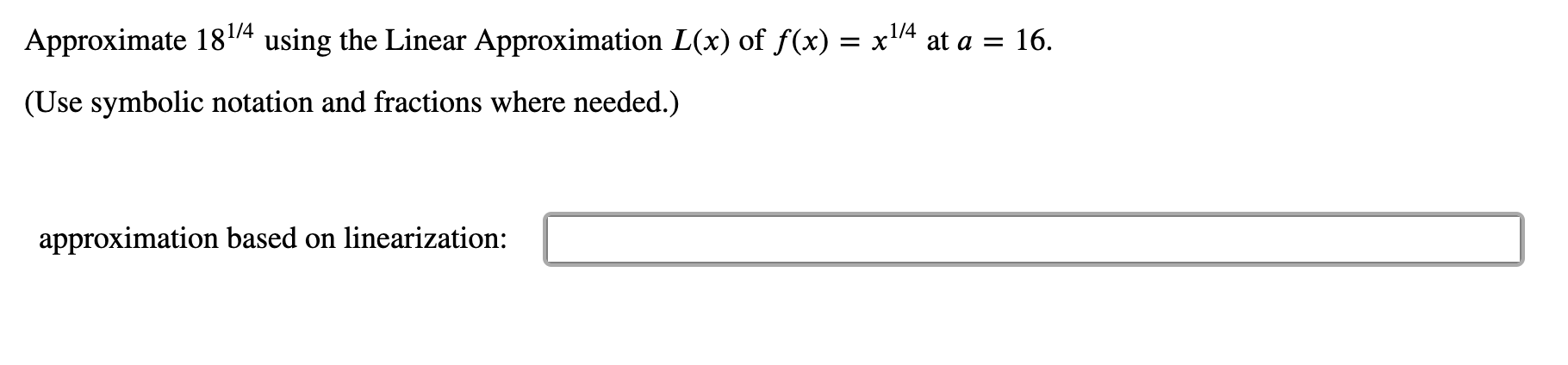# 1/4using the Linear Approximation L(x) of f(x) x14 at a = 16Approximate 181/4(Use symbolic notation and fractions where needed.)approximation based on linearization:

Questionhelp_outlineImage Transcriptionclose1/4 using the Linear Approximation L(x) of f(x) x14 at a = 16 Approximate 181/4 (Use symbolic notation and fractions where needed.) approximation based on linearization: fullscreen
check_circleExpert Solution
Step 1
Step 2
Step 3

### Want to see the full answer?

See Solution

#### Want to see this answer and more?

Solutions are written by subject experts who are available 24/7. Questions are typically answered within 1 hour*

See Solution
*Response times may vary by subject and question
Tagged in

### Other